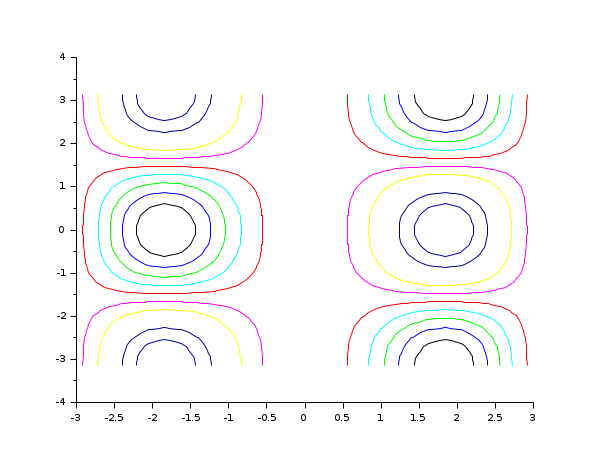Scilab Home page | Wiki | Bug tracker | Forge | Mailing list archives | ATOMS | File exchange
Change language to: Français - Português - 日本語 - Русский
Scilab Help >> Graphics > 3d_plot > contour

# contour

level curves on a 3D surface

### Syntax

```contour(x,y,z,nz,[theta,alpha,leg,flag,ebox,zlev])
contour(x,y,z,nz,<opt_args>)```

### Arguments

x,y

two real row vectors of size n1 and n2.

z

real matrix of size (n1,n2), the values of the function or a Scilab function which defines the surface `z=f(x,y)`.

nz

the level values or the number of levels.

-

If `nz` is an integer, its value gives the number of level curves equally spaced from zmin to zmax as follows:

`z= zmin + (1:nz)*(zmax-zmin)/(nz+1)`Note that the `zmin` and `zmax` levels are not drawn (generically they are reduced to points) but they can be added with

```[im,jm] = find(z == zmin);     // or zmax
plot2d(x(im)',y(jm)',-9,"000")```
-

If `nz` is a vector, `nz(i)` gives the value of the ith level curve. Note that it can be useful in order to see `zmin` and `zmax` level curves to add an epsilon tolerance: `nz=[zmin+%eps,..,zmax-%eps]`.

<opt_args>

a sequence of statements `key1=value1, key2=value2`, ... where keys may be `theta`,`alpha`,`leg`,`flag`, `ebox`,`zlev` (see below). In this case, the order has no special meaning.

theta, alpha

real values giving in degree the spherical coordinates of the observation point.

leg

string defining the captions for each axis with @ as a field separator, for example "X@Y@Z".

flag

a real vector of size three `flag=[mode,type,box]`.

mode

string representation mode.

mode=0:

the level curves are drawn on the surface defined by (x,y,z).

mode=1:

the level curves are drawn on a 3D plot and on the plan defined by the equation z=zlev.

mode=2:

the level curves are drawn on a 2D plot.

type

an integer (scaling).

type=0

the plot is made using the current 3D scaling (set by a previous call to `param3d`, `plot3d`, `contour` or `plot3d1`).

type=1

rescales automatically 3d boxes with extreme aspect ratios, the boundaries are specified by the value of the optional argument `ebox`.

type=2

rescales automatically 3d boxes with extreme aspect ratios, the boundaries are computed using the given data.

type=3

3d isometric with box bounds given by optional `ebox`, similarly to `type=1`

type=4

3d isometric bounds derived from the data, similarly to `type=2`

type=5

3d expanded isometric bounds with box bounds given by optional `ebox`, similarly to `type=1`

type=6

3d expanded isometric bounds derived from the data, similarly to `type=2`

box

an integer (frame around the plot).

box=0

nothing is drawn around the plot.

box=1

unimplemented (like box=0).

box=2

only the axes behind the surface are drawn.

box=3

a box surrounding the surface is drawn and captions are added.

box=4

a box surrounding the surface is drawn, captions and axes are added.

ebox

used when `type` in `flag` is 1. It specifies the boundaries of the plot as the vector `[xmin,xmax,ymin,ymax,zmin,zmax]`.

zlev

real number.

### Description

`contour` draws level curves of a surface z=f(x,y). The level curves are drawn on a 3D surface. The optional arguments are the same as for the function `plot3d` (except `zlev`) and their meanings are the same. They control the drawing of level curves on a 3D plot. Only `flag(1)=mode` has a special meaning.

mode=0

the level curves are drawn on the surface defined by (x,y,z).

mode=1

the level curves are drawn on a 3D plot and on the plan defined by the equation z=zlev.

mode=2

the level curves are drawn on a 2D plot.

You can change the format of the floating point number printed on the levels by using `xset("fpf",string)` where `string` gives the format in C format syntax (for example `string="%.3f"`). Use `string=""` to switch back to default format and Use `string=" "` to suppress printing.

Usually we use `contour2d` to draw levels curves on a 2D plot.

Enter the command `contour()` to see a demo.

### Examples

```t=linspace(-%pi,%pi,30);
function z=my_surface(x, y),z=x*sin(x)^2*cos(y),endfunction
contour(t,t,my_surface,10)``````t=linspace(-%pi,%pi,30);
// changing the format of the printing of the levels
xset("fpf","%.1f")
clf()
function z=my_surface(x, y),z=x*sin(x)^2*cos(y),endfunction
contour(t,t,my_surface,10)``````// 3D
t=linspace(-%pi,%pi,30);
function z=my_surface(x, y),z=x*sin(x)^2*cos(y),endfunction
z=feval(t,t,my_surface);
plot3d(t,t,z);contour(t,t,z+0.2*abs(z),20,flag=[0 2 4]);```